# Suppose that the two components operate independently and have identical performance characteristics. Let X and Y...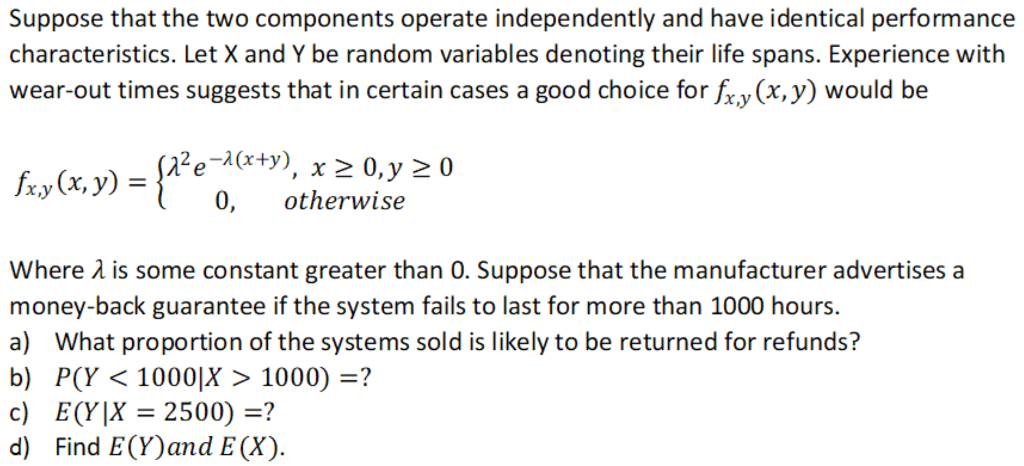Suppose that the two components operate independently and have identical performance characteristics. Let X and Y be random variables denoting their life spans. Experience with wear-out times suggests that in certain cases a good choice for fx,y(x, y) would be fxx (x, y) = {?? 122e-1(x+y), x > 0,y>0 otherwise Where 2 is some constant greater than 0. Suppose that the manufacturer advertises a money-back guarantee if the system fails to last for more than 1000 hours. a) What proportion of the systems sold is likely to be returned for refunds? b) P(Y < 1000|X > 1000) =? c) E(Y|X = 2500) =? d) Find E(Y)and E(X).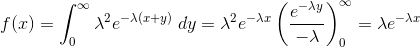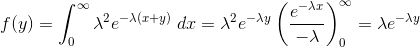d)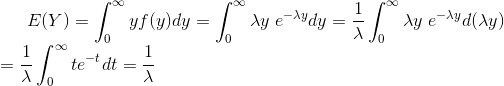where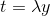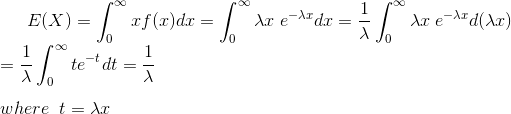a)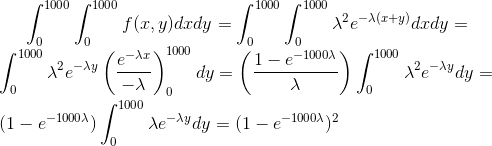b)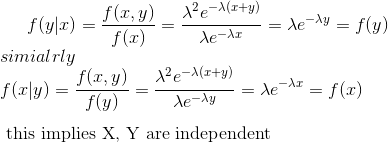so,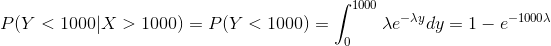c)

similarly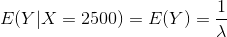##### Add Answer to: Suppose that the two components operate independently and have identical performance characteristics. Let X and Y...
Similar Homework Help Questions
• ### Suppose X and Y are two continuous random variables with probability density functions: fx(x)1 for 1<x2, fx(x) 0 otherwise, and fr (v) 3e3y for y>0, fr (y) 0 otherwise. a) Suppose X and Y ar...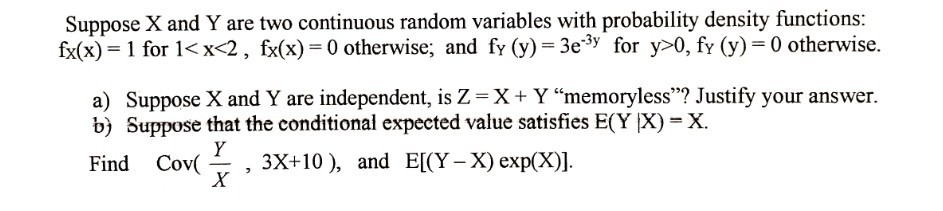Suppose X and Y are two continuous random variables with probability density functions: fx(x)1 for 1<x2, fx(x) 0 otherwise, and fr (v) 3e3y for y>0, fr (y) 0 otherwise. a) Suppose X and Y are independent, is Z-X+ Y"memoryless"? Justify your answer. b) Suppose that the conditional expected value satisfies E(Y X)-X. Find Cov0), and El(Y-X) expX)]. Suppose X and Y are two continuous random variables with probability density functions: fx(x)1 for 10, fr (y) 0 otherwise. a) Suppose X...

• ### Suppose X and Y have joint probability density function fX,Y(x,y)=70e?3x?7y for 0<x<y; and fX,Y(x,y)=0 otherwise. Find...

Suppose X and Y have joint probability density function fX,Y(x,y)=70e?3x?7y for 0<x<y; and fX,Y(x,y)=0 otherwise. Find E(X). (You may either use the joint density given here,

• ### 4. Suppose X and Y have the joint pdf f(x,y) = 6x, 0 < x <...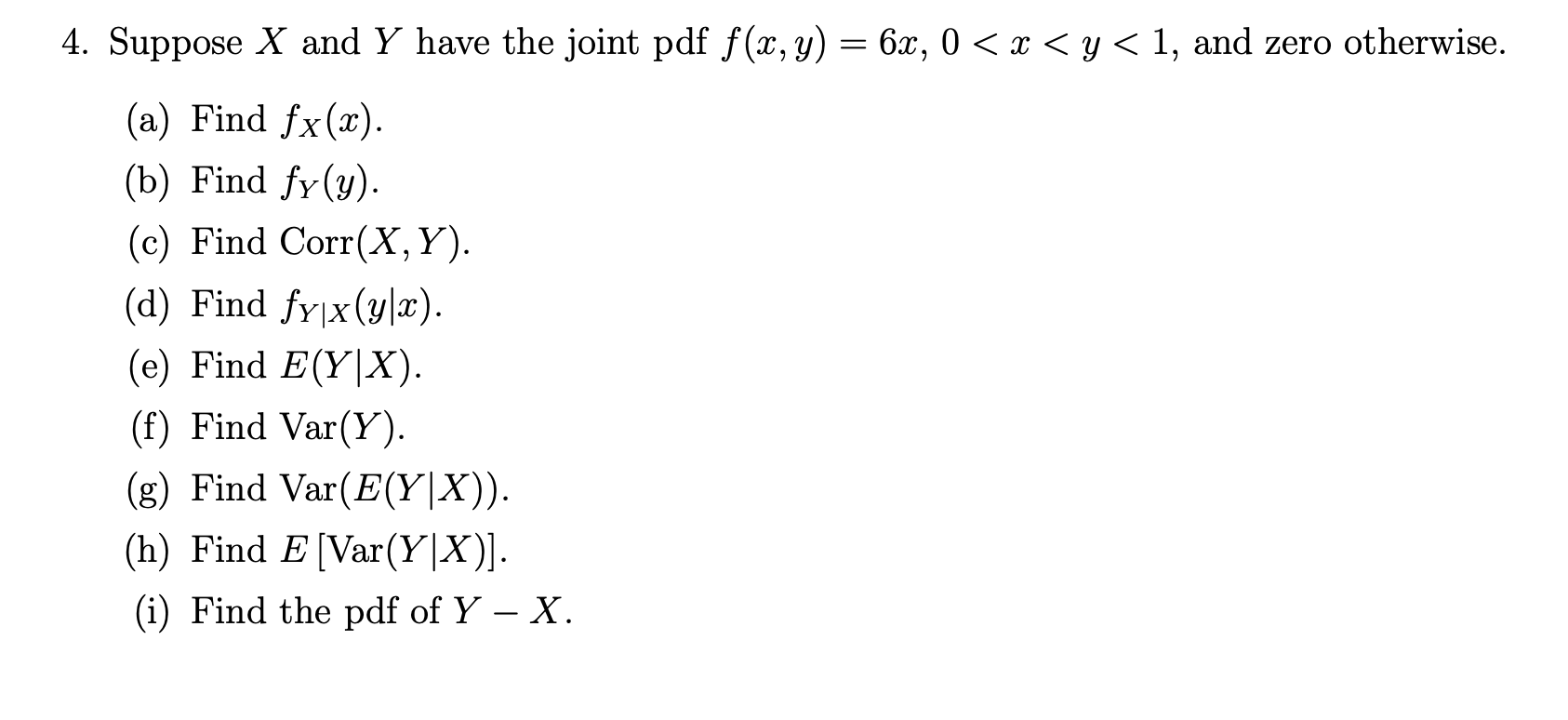4. Suppose X and Y have the joint pdf f(x,y) = 6x, 0 < x < y < 1, and zero otherwise. (a) Find fx(x). (b) Find fy(y). (c) Find Corr(X,Y). (d) Find fy x(y|x). (e) Find E(Y|X). (f) Find Var(Y). (g) Find Var(E(Y|X)). (h) Find E (Var(Y|X)]. (i) Find the pdf of Y - X.

• ### 4. Suppose that X and Y have the following joint PDF: e-(z+y) fx,Y(x,y) = :x>0, y...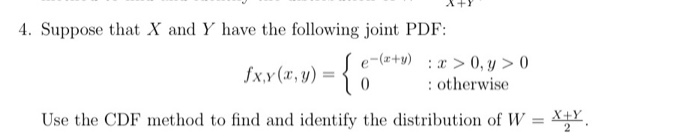4. Suppose that X and Y have the following joint PDF: e-(z+y) fx,Y(x,y) = :x>0, y > 0 : otherwise Use the CDF method to find and identify the distribution of WX

• ### . Jan wins, ue OTCS LICO. 6.127 Consider two identical electrical components. Let X and Y denote the respective lifetim...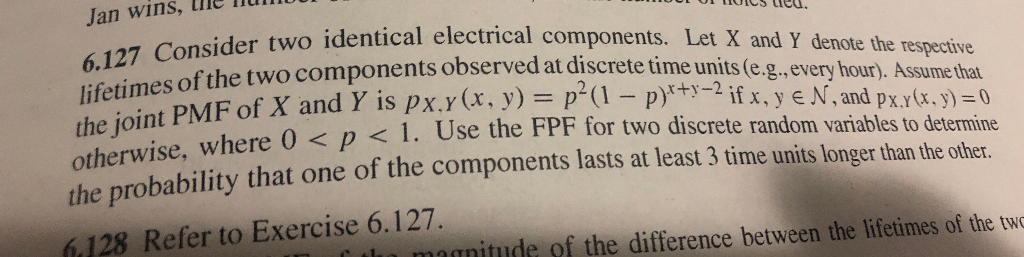. Jan wins, ue OTCS LICO. 6.127 Consider two identical electrical components. Let X and Y denote the respective lifetimes of the two components observed at discrete time units (e.g.,every hour). Assume that the joint PMF of X and Y is px.y(x, y) = p (1 - p)*+-2 if x, y e N, and px.Y(x, y) 0 otherwise, where O <p< l. Use the FPF for two discrete random variables to determine the probability that one of the components lasts...

• ### Two agents have identical quasilinear preferences U(x, y)-u(x) + y, where , x , x E...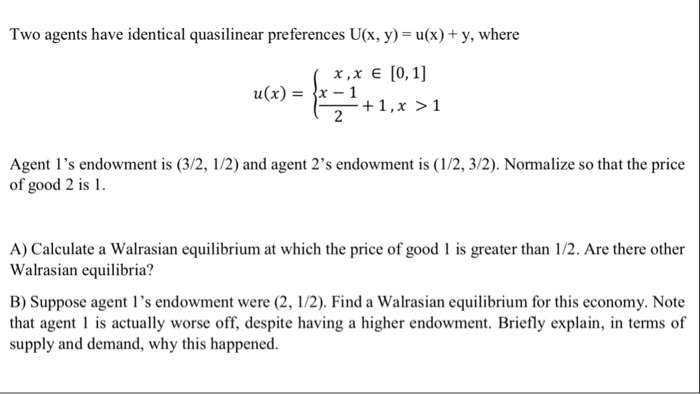Two agents have identical quasilinear preferences U(x, y)-u(x) + y, where , x , x E [0,1] Agent 1's endowment is (3/2, 1/2) and agent 2's endowment is (1/2, 3/2). Normalize so that the price of good 2 is A) Calculate a Walrasian equilibrium at which the price of good 1 is greater than 1/2. Are there other Walrasian equilibria? B) Suppose agent 1's endowment were (2, 1/2). Find a Walrasian equilibrium for this economy. Note that agent is actually...

• ### 1. Suppose that random variables X and Y are independent and have the following properties: E(X)...

1. Suppose that random variables X and Y are independent and have the following properties: E(X) = 5, Var(X) = 2, E(Y ) = −2, E(Y 2) = 7. Compute the following. (a) E(X + Y ). (b) Var(2X − 3Y ) (c) E(X2 + 5) (d) The standard deviation of Y . 2. Consider the following data set: �x = {90, 88, 93, 87, 85, 95, 92} (a) Compute x¯. (b) Compute the standard deviation of this set. 3....

• ### 5. Suppose X has the Rayleigh density otherwise 0, a. Find the probability density function for Y...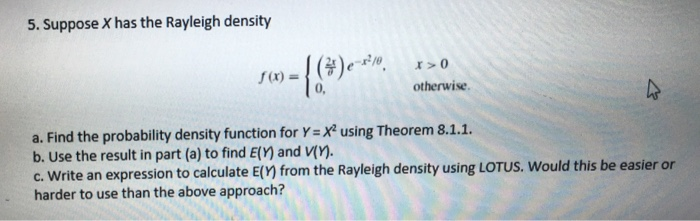5. Suppose X has the Rayleigh density otherwise 0, a. Find the probability density function for Y-X using Theorem 8.1.1. b. Use the result in part (a) to find E() and V(). c. Write an expression to calculate E(Y) from the Rayleigh density using LOTUS. Would this be easier or harder to use than the above approach? of variables in one dimension). Let X be s Y(X), where g is differentiable and strictly incr 1 len the PDF of Y...

Free Homework App